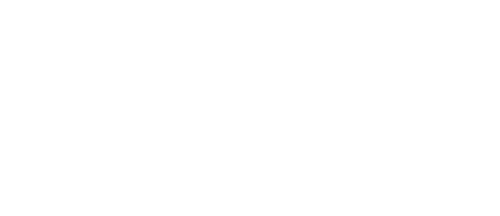Apply Now!### MATH218M - Introduction to Linear Algebra (4-0-4)

This course covers the linear systems of equations, matrix operations, determinants, linear dependency, vector space, linear transformations, eigenvalues and orthogonality. Proofs by mathematical induction and contradiction are integrated into the course curriculum. Prerequisite: Satisfactory placement test scores as defined by mathematics faculty, or MATH204M with a grade of “C” or better, or permission of the instructor.